Featured Resources
Space Math @ NASA
NSO Tests 2023
- GGSO Invitational
- UTexas Regional
- MIT Invitational
- PA Regional Astro
- PA State Astro
- Solar System State
- Wichita State University
Informal Education
Education Collaborations
Web Shortcuts
Space Math @ NASA

SpaceMath@NASA introduces students to the use of mathematics in today's scientific discoveries. Through press releases and articles, Space Math explores how many kinds of mathematics skills come together in exploring the Universe.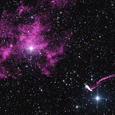A Pulsar Shot Out from a Supernova Explosion! (problem #680)Students study the speed of a pulsar ejected from a supernova explosion, and describe what would happen if the dense star collided with a star like the sun. [Grade: 6-8 | Topics: Scientific notation; speed=distance/time; unit conversions; density] (PDF) [Press Release]Exploring the Evaporating Exoplanet HD189733b (problem #561)Students estimate how quickly this planet will lose its atmosphere and evaporate at its present loss rate of 6 million tons/second [Grade: 6-8 | Topics:mass=densityx volume; rates; volume of a sphere ] (PDF) [Press Release]Giant Gas Cloud in System NGC 6240 (problem #511)Students use scientific notation and volume of sphere to estimate the density of the gas cloud, and the number of hydrogen atoms per cubic meter. [Grade: 8-10 | Topics:Volume of a sphere; scientific notation; unit conversion ] (PDF) [Press Release]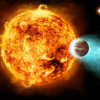Chandra Sees a Distant Planet Evaporating (problem #439)The planet CoRot2b is losing mass at a rate of 5 million tons per second. Students estimate how long it will take for the planet to lose its atmosphere.[Grade: 6-8 | Topics: Scientific Notation; RAte = Amount/Time] (PDF) [Press Release]Estimating the Size and Mass of a Black Hole (problem #417)Students use a simple formula to estimate the size of a black hole located 3.8 billion light years from Earth, recently studied by NASA's Chandra and Swift satellites. [Grade: 8-10 | Topics: distance=speed x time] (PDF) [Press Release]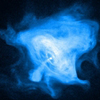The Crab Nebula - Exploring a pulsar up close! (problem #398)Students work with a photograph to determine its scale and the time taken by light and matter to reach a specified distance. [Grade: 6-8 | Topics: Scale drawings; unit conversion; distance = speed x time] (PDF) [Press Release]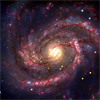X-rays from hot gases near the black hole SN1979c (problem #390) Students use two functions to estimate the size of a black hole from the gas emitting x-rays which is flowing into it. [Grade: 8-10 | Topics: Functions; substitution; evaluation] (PDF) [Press Release]Estimating the diameter of the SN1979c black hole (problem #389) Students use simple equations to learn about the various definitions for the sizes of black holes in terms of their event horizons, last photon orbit, and last stable particle orbit radii, and apply this to the recently discovered 'baby' black hole in the galaxy M-100. [Grade: 6-8 | Topics: evaluating linear functions; integer math; metric units] (PDF) [Press Release]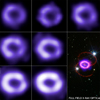Chandra Studies an Expanding Supernova Shell (problem #314) Using a millimeter ruler and a sequence of images of a gaseous shell between 2000 and 2005, students calculate the speed of the material ejected by Supernova 1987A. [Grade: 6-9 | Topics: Measuring; Metric Units; speed=distance/time] (PDF) [Press Release]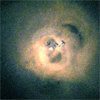Chandra Spies the Longest Sound Wave in the Universe (problem #289)Students use an image of sound waves produced by a massive black hole to determine wavelength, and comparisons with musical scale to find how many octaves this sound wave is below the wavelength of middle-C. [Grade: 6-8 | Topics: metric measurement; scaling; Scientific Notation; exponents] (PDF) [Press Release]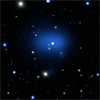Chandra Sees the Most Distant Cluster in the Universe (problem #285) Students work with kinetic energy and escape velocity to determine the mass of a distant cluster of galaxies by using information about its x-ray light emissions. [Grade: 9-12 | Topics: Algebra I; Solving for X; Scientific notation] (PDF) [Press Release]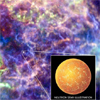Chandra Observatory Sees the Atmosphere of a Neutron Star (problem #283) Students determine the mass of the carbon atmosphere of the neutron star Cas-A. [Grade: 8-10 | Topics: Volume of spherical shell; mass = density x volume] (PDF) [Press Release]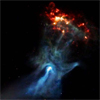The Hand of Chandra (problem #234) Students use an image from the Chandra Observatory to measure a pulsar ejecting a cloud of gas. [Grade: 6-8 | Topics: Scientific Notation; proportions; angle measure] (PDF) [Press Release]Exploring Angular Size (problem #144) Students examine the concept of angular size and how it relates to the physical size of an object and its distance. A Chandra Satellite x-ray image of the star cluster NGC-6266 is used, along with its distance, to determine how far apart the stars are based on their angular separations. [Grade: 7 - 10 | Topics: Scientific Notation; degree measurement; physical size=distance x angular size.] (PDF) [Press Release]